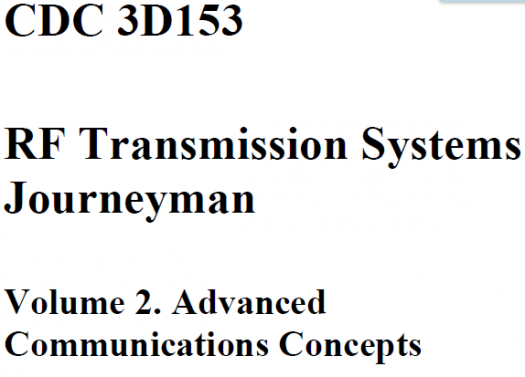# 3D153 Vol 2 Ure

100 Questions | Total Attempts: 105Settings.

• 1.
(201) Equipment used for generating, amplifying, and transmitting RF carrier is collectively called
• A.

• B.

An antenna

• C.

A transmitter

• D.

A transmission line

• 2.
(201) What basic receiver function involves having the transmitted electromagnetic wave passthrough the receive antenna in such a manner as to induce a voltage in the antenna?
• A.

Reproduction

• B.

Reception

• C.

Detection

• D.

Selection

• 3.
(201) The ability of a receiver to reproduce the signal of a very weak station is the characteristic ofthe receiver’s
• A.

Fidelity

• B.

Capacity

• C.

Sensitivity

• D.

Selectivity

• 4.
(201) The ability to reproduce the input signal accurately is the characteristics of the receiver’s
• A.

Fidelity

• B.

Capacity

• C.

Sensitivity

• D.

Fidelity

• 5.
(202) Which of the following CANNOT be performed by the transceiver’s computerizedcomponents and micro-circuitry?
• A.

Specific equipment faults.

• B.

Internal equipment tests

• C.

Internal calibrations

• D.

Repair itself

• 6.
(203) Resistance of the conductor material in a transmission line leads to which type of loss?
• A.

Copper

• B.

• C.

Inductive

• D.

Skin-effect

• 7.
(203) Leakage loss in a transmission line can be minimized by using a
• A.

Thinner conductor

• B.

Thicker conductor

• C.

Very low-resistance dielectric

• D.

Very high-resistance dielectric

• 8.
(203) A transmission line that consists of a center conductor placed inside a rigid metal tubefunctioning as the outer tube is called a
• A.

Flexible coaxial cable.

• B.

Rigid coaxial cable.

• C.

Waveguide

• D.

• 9.
(203) Which statement concerning waveguides is FALSE?
• A.

Very slight damage to the external surface of a waveguide can cause arcing.

• B.

Their conductive material construction is easily dented.

• C.

Effectiveness of waveguides is hindered by moisture.

• D.

Waveguides can be destroyed by corrosion.

• 10.
(203) What two properties of a transmission line determine its characteristic impedance?
• A.

Inductance and resistance.

• B.

Inductance and capacitance.

• C.

Resistance and capacitance.

• D.

Length and type of shielding.

• 11.
(203) Using figure 1–10, determine the electrical length if the frequency is changed from 150MHz to 250 MHz.
• A.

8.33 meters

• B.

8.33 wavelengths

• C.

83.3 meters

• D.

83.3 wavelengths

• 12.
(203) Which statement concerning line lengths is TRUE?
• A.

Frequency and electrical length are inversely proportional.

• B.

Frequency and physical length are directly proportional.

• C.

With constant physical length, frequency and electrical length are directly related.

• D.

With constant physical length, frequency and electrical length are inversely related.

• 13.
(203) A nonresonant transmission line is a line
• A.

Having reflected waves.

• B.

Having no reflected waves.

• C.

With maximum voltage across its open termination.

• D.

With maximum voltage across its shorted termination.

• 14.
(203) If a transmission line is terminated in an open, what will likely result?
• A.

Signal loss would be negligible.

• B.

There would be significant signal loss.

• C.

Current would be at maximum at the termination.

• D.

Voltage would be at minimum at the termination.

• 15.
(204) The concept that alternating current changes in magnitude and reverses its direction duringeach cycle is
• A.

An unproven hypothesis

• B.

The definition of propagation.

• C.

• D.

What led to the discovery of direct current.

• 16.
(204) At what point do magnetic fields around a wire no longer have time to collapse completelybetween alternations?
• A.

60 cycles per second (cps).

• B.

120 cps

• C.

10,000 cps

• D.

15,000 cps

• 17.
(204) Which basic field detaches from the antenna and travels through space at great distances?
• A.

• B.

Induction

• C.

Gravitational

• D.

Electromotive

• 18.
(204) Areas in which no antenna radiation pattern passes through are called
• A.

Nulls

• B.

Lobes

• C.

Free space

• D.

Nonresonant

• 19.
(205) If the electric field component travels in a plane perpendicular to the Earth’s surface, theradio wave is considered to be polarized
• A.

Magnetically

• B.

Horizontally.

• C.

Circularly.

• D.

Vertically

• 20.
(206) The actual range of frequencies within the antenna’s bandwidth is called the
• A.

Wavelength

• B.

Operating zone

• C.

Frequency range

• D.

Bandwidth range

• 21.
(206) What measurement is used to determine whether an antenna is resonant at a particularfrequency?
• A.

Distortion.

• B.

Standing wave ratio.

• C.

Signal-to-noise ratio.

• D.

Percent of modulation.

• 22.
(206) The standard used to measure the radiating effectiveness (gain) of an antenna system is the
• A.

Marconi antenna.

• B.

Isotropic antenna.

• C.

Hertz antenna.

• D.

Whip antenna.

• 23.
(206) What does the overall effectiveness of an entire transmitting and receiving system dependlargely upon?
• A.

Impedance matching.

• B.

Antenna distance.

• C.

Antenna properties.

• D.

Line characteristics.

• 24.
(206) The ability of an antenna to both receive and transmit equally well is known as theantenna’s
• A.

Bandwidth.

• B.

Resonance.

• C.

Reciprocity.

• D.

Effectiveness.

• 25.
• A.

Omni-directional.

• B.

Uni-directional.

• C.

Bi-directional.

• D.

Directional.

Related TopicsBack to top